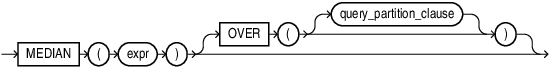## MEDIAN

SyntaxDescription of the illustration median.eps

for information on syntax, semantics, and restrictions

Purpose

`MEDIAN` is an inverse distribution function that assumes a continuous distribution model. It takes a numeric or datetime value and returns the middle value or an interpolated value that would be the middle value once the values are sorted. Nulls are ignored in the calculation.

This function takes as arguments any numeric data type or any nonnumeric data type that can be implicitly converted to a numeric data type. If you specify only `expr`, then the function returns the same data type as the numeric data type of the argument. If you specify the `OVER` clause, then Oracle Database determines the argument with the highest numeric precedence, implicitly converts the remaining arguments to that data type, and returns that data type.

Table 2-8 for more information on implicit conversion and for information on numeric precedence

The result of `MEDIAN` is computed by first ordering the rows. Using `N` as the number of rows in the group, Oracle calculates the row number (`RN`) of interest with the formula `RN` = (`1` + (0.`5`*(`N`-`1`)). The final result of the aggregate function is computed by linear interpolation between the values from rows at row numbers `CRN` = `CEILING`(`RN`) and `FRN` = `FLOOR`(`RN`).

The final result will be:

```   if (CRN = FRN = RN) then
(value of expression from row at RN)
else
(CRN - RN) * (value of expression for row at FRN) +
(RN - FRN) * (value of expression for row at CRN)
```

You can use `MEDIAN` as an analytic function. You can specify only the `query_partition_clause` in its `OVER` clause. It returns, for each row, the value that would fall in the middle among a set of values within each partition.

Compare this function with these functions:

• PERCENTILE_CONT, which returns, for a given percentile, the value that corresponds to that percentile by way of interpolation. `MEDIAN` is the specific case of `PERCENTILE_CONT` where the percentile value defaults to 0.5.

• PERCENTILE_DISC, which is useful for finding values for a given percentile without interpolation.

Aggregate Example

The following query returns the median salary for each department in the `hr.employees` table:

```SELECT department_id, MEDIAN(salary)
FROM employees
GROUP BY department_id
ORDER BY department_id;

DEPARTMENT_ID MEDIAN(SALARY)
------------- --------------
10           4400
20           9500
30           2850
40           6500
50           3100
60           4800
70          10000
80           8900
90          17000
100           8000
110          10154
7000```

Analytic Example

The following query returns the median salary for each manager in a subset of departments in the `hr.employees` table:

```SELECT manager_id, employee_id, salary,
MEDIAN(salary) OVER (PARTITION BY manager_id) "Median by Mgr"
FROM employees
WHERE department_id > 60
ORDER BY manager_id, employee_id;

MANAGER_ID EMPLOYEE_ID     SALARY Median by Mgr
---------- ----------- ---------- -------------
100         101      17000         13500
100         102      17000         13500
100         145      14000         13500
100         146      13500         13500
100         147      12000         13500
100         148      11000         13500
100         149      10500         13500
101         108      12008         12008
101         204      10000         12008
101         205      12008         12008
108         109       9000          7800
108         110       8200          7800
108         111       7700          7800
108         112       7800          7800
108         113       6900          7800
145         150      10000          8500
145         151       9500          8500
145         152       9000          8500
. . .```